6.4.1 Step 1: Search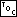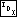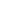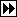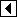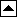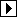The first thing to do is to search for the point to insert the new node. In a manner similar to AVL deletion, we keep a stack of nodes tracking the path followed to arrive at the insertion point, so that later we can move up the tree in rebalancing.

```199. <Step 1: Search RB tree for insertion point 199> =
pa = (struct rb_node *) &tree->rb_root;
da = 0;
k = 1;
for (p = tree->rb_root; p != NULL; p = p->rb_link[da[k - 1]])   {
int cmp = tree->rb_compare (item, p->rb_data, tree->rb_param);
if (cmp == 0)
return &p->rb_data;

pa[k] = p;
da[k++] = cmp > 0;
}
```

This code is included in 197 and 210.